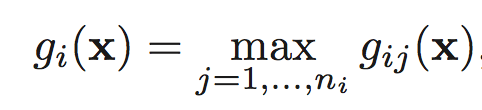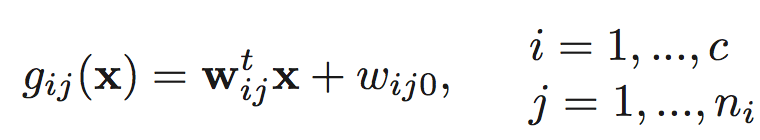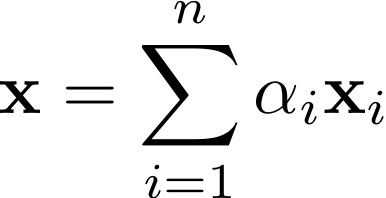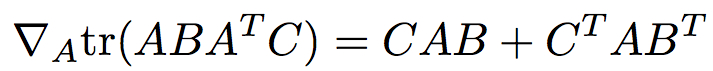# HW1 Decision Tree, Linear Regression

Make sure you check the syllabus for the due date. Please use the notations adopted in class, even if the problem is stated in the book using a different notation.

We are not looking for very long answers (if you find yourself writing more than one or two pages of typed text per problem, you are probably on the wrong track). Try to be concise; also keep in mind that good ideas and explanations matter more than exact details.

Submit all code files Dropbox (create folder HW1 or similar name). Results can be pdf or txt files, including plots/tabels if any.

"Paper" exercises: submit using Dropbox as pdf, either typed or scanned handwritten.

### DATASET 1: Housing data, training and testing sets (description). The last column are the labels.

DATATSET 2: Spambase dataset available from the UCI Machine Learning Repository.

You can try to normalize each column (feature) separately with wither one of the following ideas. Do not normalize labels.

• Shift-and-scale normalization: substract the minimum, then divide by new maximum. Now all values are between 0-1
• Zero mean, unit variance : substract the mean, divide by the appropriate value to get variance=1.
• When normalizing a column (feature), make sure to normalize its values across all datapoints (train,test,validation, etc)

The Housing dataset comes with predefined training and testing sets. For the Spambase dataset use K-fold cross-validation :

• split into K folds
• run your algorithm K times each time training on K-1 of them and testing on the remaining one
• average the error across the K runs.

### PROBLEM 1 [50 points]

Using each dataset, build a decision tree (or regression tree) from the training set. Since the features are numeric values, you will need to use thresholds mechanisms. Report (txt or pdf file) for each dataset the training and testing error for each of your trials:

• simple decision tree using something like Information Gain or other Entropy-like notion of randomness
• regression tree
• try to limit the size of the tree to get comparable training and testing errors (avoid overfitting typical of deep trees)

### PROBLEM 2 [50 points]

Using each of the two datasets above, apply regression on the training set to find a linear fit with the labels.  Implement linear algebra exact solution (normal equations).

• Compare the training and testing errors (mean sum of square differences between prediction and actual label).
• Compare with the decision tree results

### PROBLEM 3 [20 points]

DHS chapter8, Pb1. Given an arbitrary decision tree, it might have repeated queries splits (feature f, threshold t) on some paths root-leaf. Prove that there exists an equivalent decision tree only with distinct splits on each path.

DHS chapter8,
a) Prove that for any arbitrary tree, with possible unequal branching ratios throughout, there exists a binary tree that implements the same classification functionality.
b) Consider a tree with just two levels  - a root node  connected to B leaf nodes (B>=2) . What are  then upper and the lower limits on the number of levels in a functionally equivalent binary tree, as a function of B?
c)  As in b), what are the upper and lower limits on number of nodes in a functionally equivalent binary tree?

### PROBLEM 4 [20 points GR-ONLY]

DHS chapter8,
Consider training a binary decision tree using entropy splits.
a) Prove that the decrease in entropy by a split on a binary yes/no feature can never be greater than 1 bit.
b)  Generalize this result to the case  of arbitrary branching  B>1.

### PROBLEM 5 [20 points]

Derive explicit formulas for normal equations solution presented in class for the case of one input dimension.

(Essentially assume the data is (x_i,y_i) i=1,2,..., m and you are looking for h(x) = ax+b that realizes the minimum mean square error. The problem asks you to write down explicit formulas for a and b.)

HINT: Do not simply copy the formulas from here (but do read the article): either take the general formula derived in class and make the calculations (inverse, multiplications, transpose) for one dimension or derive the formulas for a and b from scratch; in either case show the derivations. You can compare your end formulas with the ones linked above.

### PROBLEM 6 [Extra Credit, read DHS ch5]

DHS chapter5
A classifier is said to be a piecewise linear machine if its discriminant functions have the formwhere(a) Indicate how a piecewise linear machine can be viewed in terms of a linear machine for classifying subclasses of patterns.
(b) Show that the decision regions of a piecewise linear machine can be non convex and even multiply connected.
(c) Sketch a plot of gij(x) for a one-dimensional example in which n1 = 2 and n2 = 1 to illustrate your answer to part (b)

### PROBLEM 7 [20points GR-ONLY]

DHS chapter5,
The convex hull of a set of vectors xi,i = 1,...,n is the set of all vectors of the formwhere the coefficients αi are nonnegative and sum to one. Given two sets of vectors, show that either they are linearly separable or their convex hulls intersect.
Hint on easy part: that the two conditions cannot happen simultaneously. Suppose that both statements are true, and consider the classification of a point in the intersection of the convex hulls.
[Extra credit] Hard part: that at least one of the two conditions must hold. Suppose that the convex hulls dont intersect; then show the points are linearly separable.

### PROBLEM 8 [ExtraCredit]

With the notation used in class (and notes), prove that